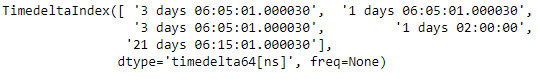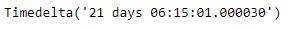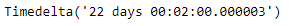# Python | Pandas TimedeltaIndex.min

• Last Updated : 28 Dec, 2018

Python is a great language for doing data analysis, primarily because of the fantastic ecosystem of data-centric python packages. Pandas is one of those packages and makes importing and analyzing data much easier.

Pandas` TimedeltaIndex.min()` function return the minimum value of the TimedeltaIndex object or minimum along an axis.

Syntax : TimedeltaIndex.min(axis=None, *args, **kwargs)

Parameters : None

Return : Timedelta object

Example #1: Use `TimedeltaIndex.min()` function to find the minimum value in the given TimedeltaIndex object.

 `# importing pandas as pd``import` `pandas as pd`` ` `# Create the TimedeltaIndex object``tidx ``=` `pd.TimedeltaIndex(data ``=``[``'3 days 06:05:01.000030'``, ``'1 days 06:05:01.000030'``,``                                ``'3 days 06:05:01.000030'``, ``'1 days 02:00:00'``, ``                                                 ``'21 days 06:15:01.000030'``])`` ` `# Print the TimedeltaIndex object``print``(tidx)`

Output :Now we will use the `TimedeltaIndex.min()` function to find the minimum value in tidx object.

 `# find minimum value``tidx.``min``()`

Output :As we can see in the output, the `TimedeltaIndex.min()` function has returned the minimum value in the given TimedeltaIndex object.

Example #2: Use `TimedeltaIndex.min()` function to find the minimum value in the given TimedeltaIndex object.

 `# importing pandas as pd``import` `pandas as pd`` ` `# Create the TimedeltaIndex object``tidx ``=` `pd.TimedeltaIndex(data ``=``[``'06:05:01.000030'``, ``'3 days 06:05:01.000030'``,``                                ``'22 day 2 min 3us 10ns'``, ``'+23:59:59.999999'``,``                             ``'13 days 06:05:01.000030'``, ``'+12:19:59.999999'``])`` ` `# Print the TimedeltaIndex object``print``(tidx)`

Output :Now we will use the `TimedeltaIndex.min()` function to find the minimum value in tidx object.

 `# find minimum value``tidx.``min``()`

Output :As we can see in the output, the `TimedeltaIndex.min()` function has returned the minimum value in the given TimedeltaIndex object.

My Personal Notes arrow_drop_up